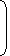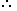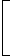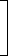# Tips and Tricks to solve aptitude problems on Mixtures and Alligation1. Alligation:It is the rule that enables us to find the ratio in which two or more ingredients at the given price must be mixed to produce a mixture of desired price.
2. Mean Price:The cost of a unit quantity of the mixture is called the mean price.
3. Rule of Alligation:If two ingredients are mixed, thenQuantity of cheaper=C.P. of dearer – Mean PriceQuantity of dearer Mean price – C.P. of cheaper

We present as under:

 C.P. of a unit quantity of cheaperC.P. of a unit quantity of dearer (c) Mean Price (m) (d) (d – m) (m – c)(Cheaper quantity) : (Dearer quantity) = (d – m) : (m – c).

4. Suppose a container contains x of liquid from which y units are taken out and replaced by water.
 After n operations, the quantity of pure liquid =x1 – ynunits.

Correct! Wrong!

Correct! Wrong!

Correct! Wrong!

Correct! Wrong!

#### 5.How many kilogram of sugar costing Rs. 9 per kg must be mixed with 27 kg of sugar costing Rs. 7 per kg so that there may be a gain of 10% by selling the mixture at Rs. 9.24 per kg?

Correct! Wrong!

quiz on alligation or mixture
congooo!!